﻿ 基于不同压气机特性曲线预测方法的燃气轮机建模研究
 舰船科学技术2023, Vol. 45Issue (1): 129-134    DOI: 10.3404/j.issn.1672-7649.2023.01.023PDF

Gas turbine modeling based on different compressor characteristic curve prediction methods
, HUANG Wei
College of Automation Engineering, Shanghai University of Electric Power, Shanghai 200090, China
Abstract: In order to improve the overall simulation accuracy of gas turbine model, the least square method, BP neural network method and XGboost algorithm are used to fit the compressor characteristic curve. Taking a double shaft gas turbine as the research object, the fitting results of three compressor characteristic curves are applied to the overall simulation of gas turbine.The results show that the accuracy of the XGboost and BP neural network is the highest in the prediction of compressor characteristic curve, and the least square method is relatively low; In the overall simulation of gas turbine, the three methods can achieve good accuracy in the calculation of cross-section parameters of gas turbine. The gas turbine simulation model based on XGboost algorithm has the highest calculation accuracy of gas turbine section parameters, and the highest average relative error is only 1.15%. Because the fitting accuracy of compressor characteristic curve directly affects the calculation error of gas turbine section parameters, the XGboost algorithm and BP neural network method can provide reference for improving the accuracy of gas turbine model.
Key words: gas turbine     mechanism model     compressor characteristic curve     fitting method
0 引　言

1 燃气轮机机理建模 1.1 压气机模型

 $\frac{{{G_{in}}\sqrt {{T_{in}}} }}{{{P_{in}}}} = {f_1}\left( {\frac{{{P_{out}}}}{{{P_{in}}}},\frac{n}{{\sqrt {{T_{in}}} }}} \right)，$ (1)
 ${\eta _c} = {f_2}\left( {\frac{{{P_{out}}}}{{{P_{in}}}},\frac{n}{{\sqrt {{T_{in}}} }}} \right)。$ (2)

 ${T_{out}} = {T_{in}}\left\{ {1 + \frac{{\left[ {{\text{π} _c}^{\frac{{\left( {ka - 1} \right)}}{{ka}}} - 1} \right]}}{{{\eta _c}}}} \right\}，$ (3)
 ${N_c} = \frac{{{G_{in}}{C_{pa}}{T_{in}}\left( {{\text{π}_c}^{\frac{{ka - 1}}{{ka}}} - 1} \right)}}{{{\eta _c}}} 。$ (4)

1.2 燃烧室模型

 ${T_{out}} = {T_{in}} + \frac{{{\eta _b}{H_u}{G_f}}}{{{G_{out}}{C_{pg}}}}，$ (5)
 $\frac{{{\rm{d}}{P_{out}}}}{{{\rm{d}}t}} = \frac{{{R_g}T\left( {{G_{in}} + {G_f} - {G_{out}}} \right)}}{V}。$ (6)

1.3 涡轮模型

 $\frac{{{G_T}\sqrt {{T_{in}}} }}{{{P_{in}}}} = {f_1}\left( {\frac{{{P_{in}}}}{{{P_{out}}}},\frac{n}{{\sqrt {{T_{in}}} }}} \right)，$ (7)
 ${\eta _T} = {f_2}\left( {\frac{{{P_{in}}}}{{{P_{out}}}},\frac{n}{{\sqrt {{T_{in}}} }}} \right)。$ (8)

 ${T_{out}} = {T_{in}}\left[ {1 - \left( {1 - \frac{1}{{{\text{π}_T}^{\frac{{kg - 1}}{{kg}}}}}} \right){\eta _T}} \right] ，$ (9)
 ${N_T} = {G_{in}}{C_{pg}}{T_{in}}\left[ {1 - \left( {1 - \frac{1}{{{\text{π} _T}^{\frac{{kg - 1}}{{kg}}}}}} \right){\eta _T}} \right]。$ (10)

1.4 转子模型

 $I\frac{{{\rm{d}}\omega }}{{{\rm{d}}t}} = {M_T} - {M_c} - {M_L}，$ (11)

$\omega = \dfrac{{2\text{π} n}}{{60}}$ 代入式（11）得：

 $\frac{{{\rm{d}}n}}{{{\rm{d}}t}} = \frac{{30}}{{I\text{π}}}\left( {{M_T} - {M_c} - {M_L}} \right) ，$ (12)

$P = \dfrac{M}{\omega }$ $\omega = \dfrac{{2\text{π}n}}{{60}}$ 代入式（12）得：

 $\frac{{{\rm{d}}n}}{{{\rm{d}}t}} = \frac{{900}}{{I{\text{π} ^2}n}}\left( {{P_t} - {P_c} - {P_L}} \right)，$ (13)

2 三种压气机方法拟合结果分析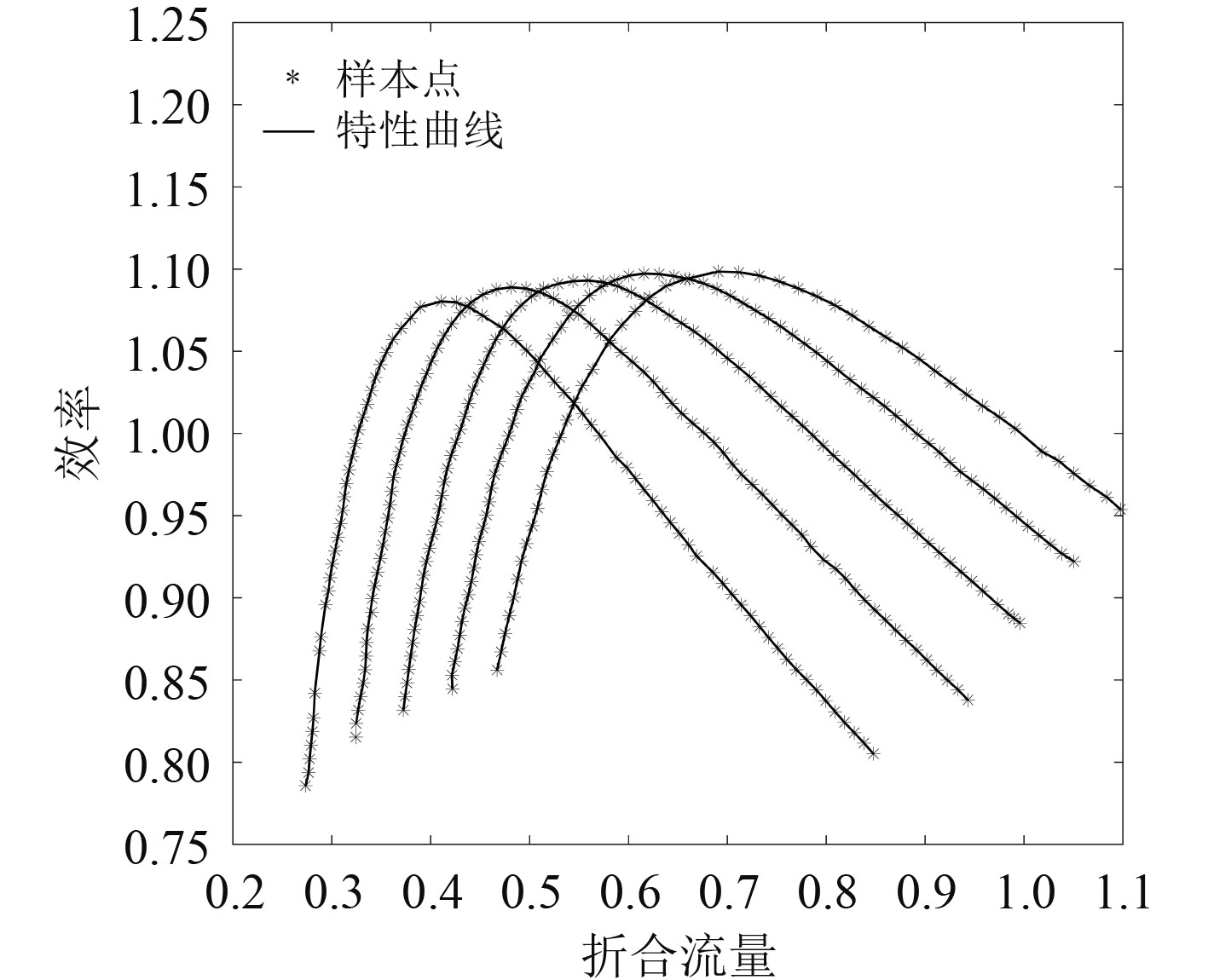图 1 压气机效率-折合流量特性曲线 Fig. 1 Compressor efficiency-flow characteristic curve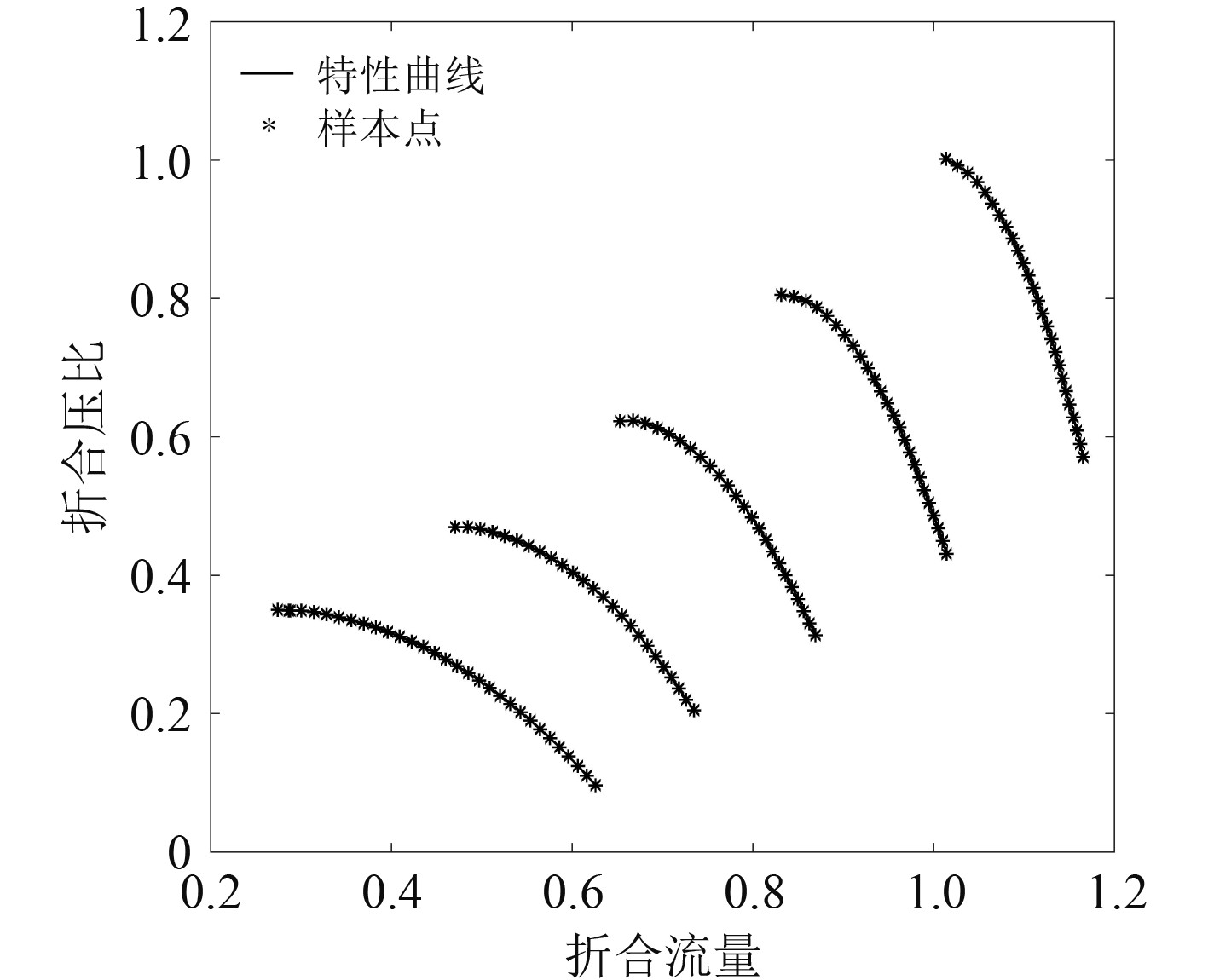图 2 压气机压比-折合流量特性曲线 Fig. 2 Compressor pressure ratio-flow characteristic curve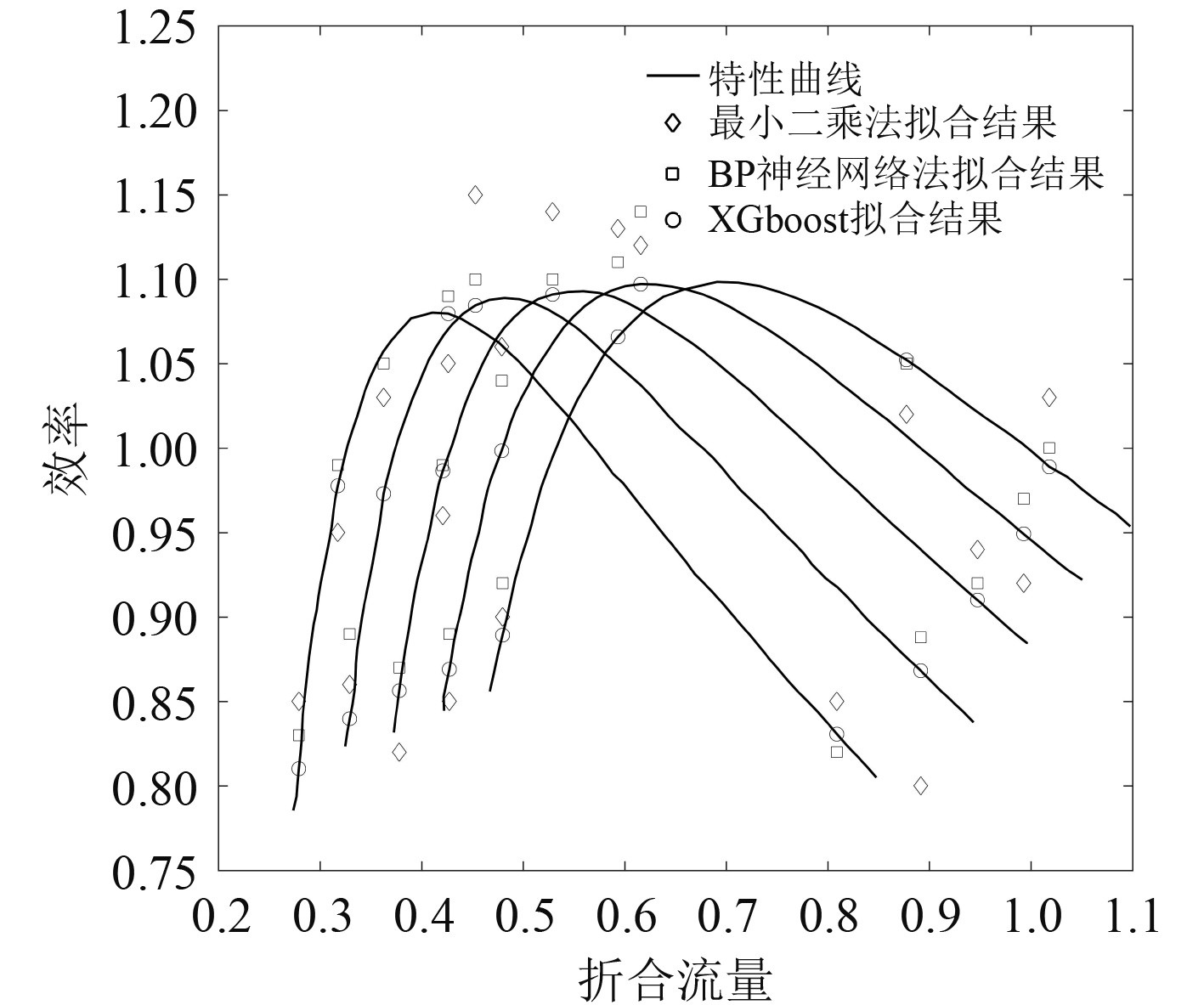图 3 效率-折合流量预测对比 Fig. 3 Comparison of efficiency-equivalent flow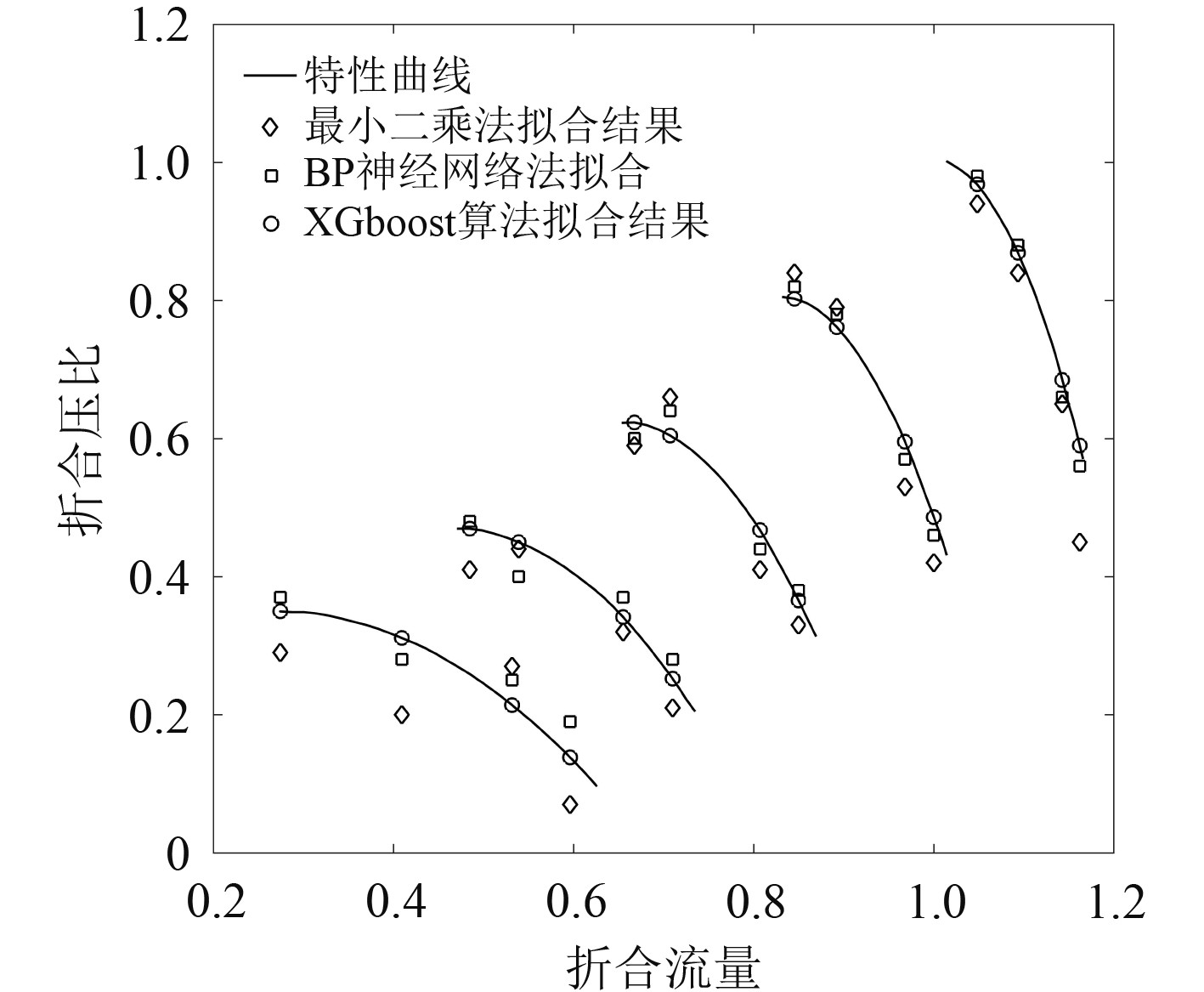图 4 压比-折合流量预测对比 Fig. 4 Comparison of pressure ratio-equivalent flow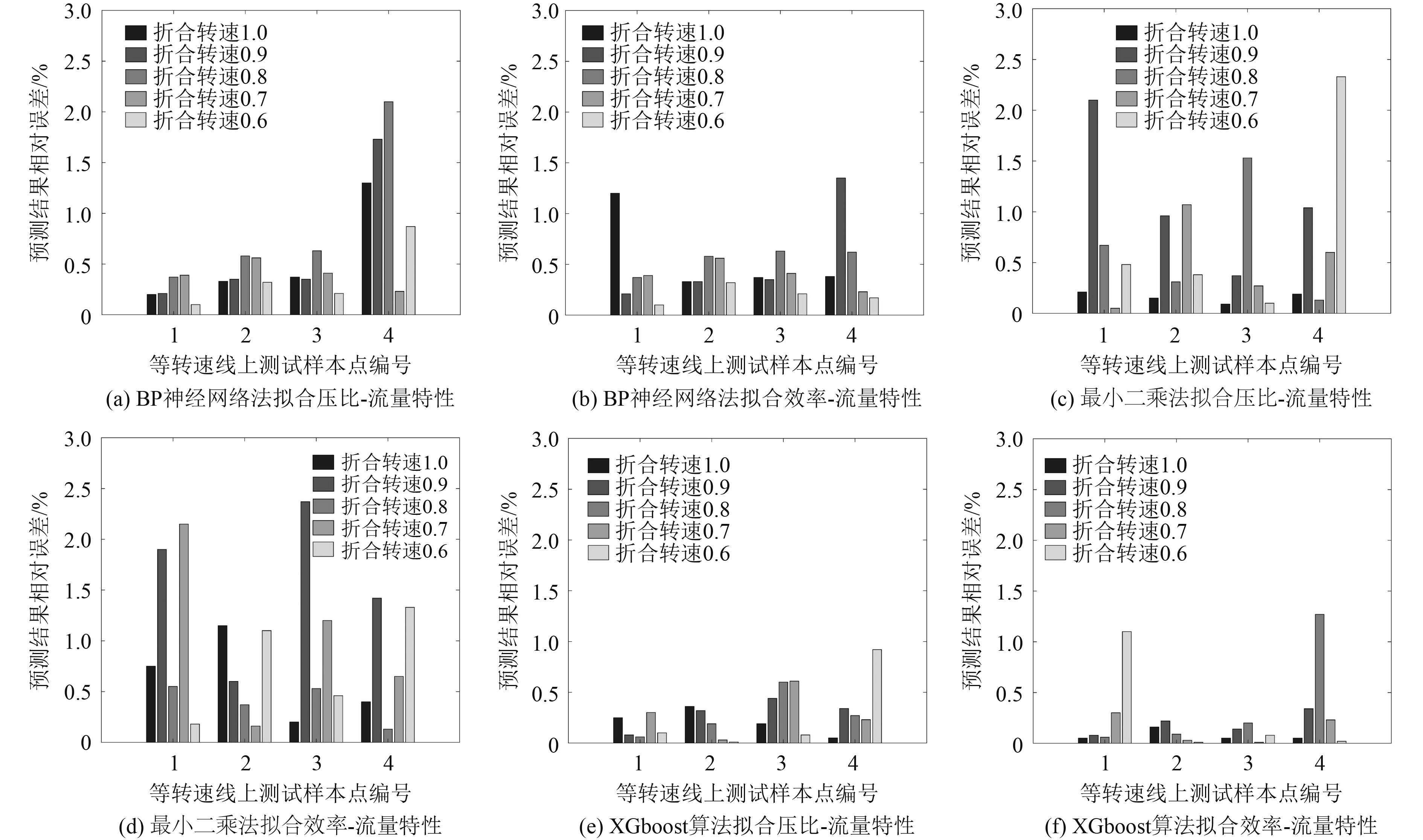图 5 3种方法预测结果的相对误差 Fig. 5 Relative error of prediction results of three methods

3 三种压气机性能预测方法在燃气轮机整体仿真中的应用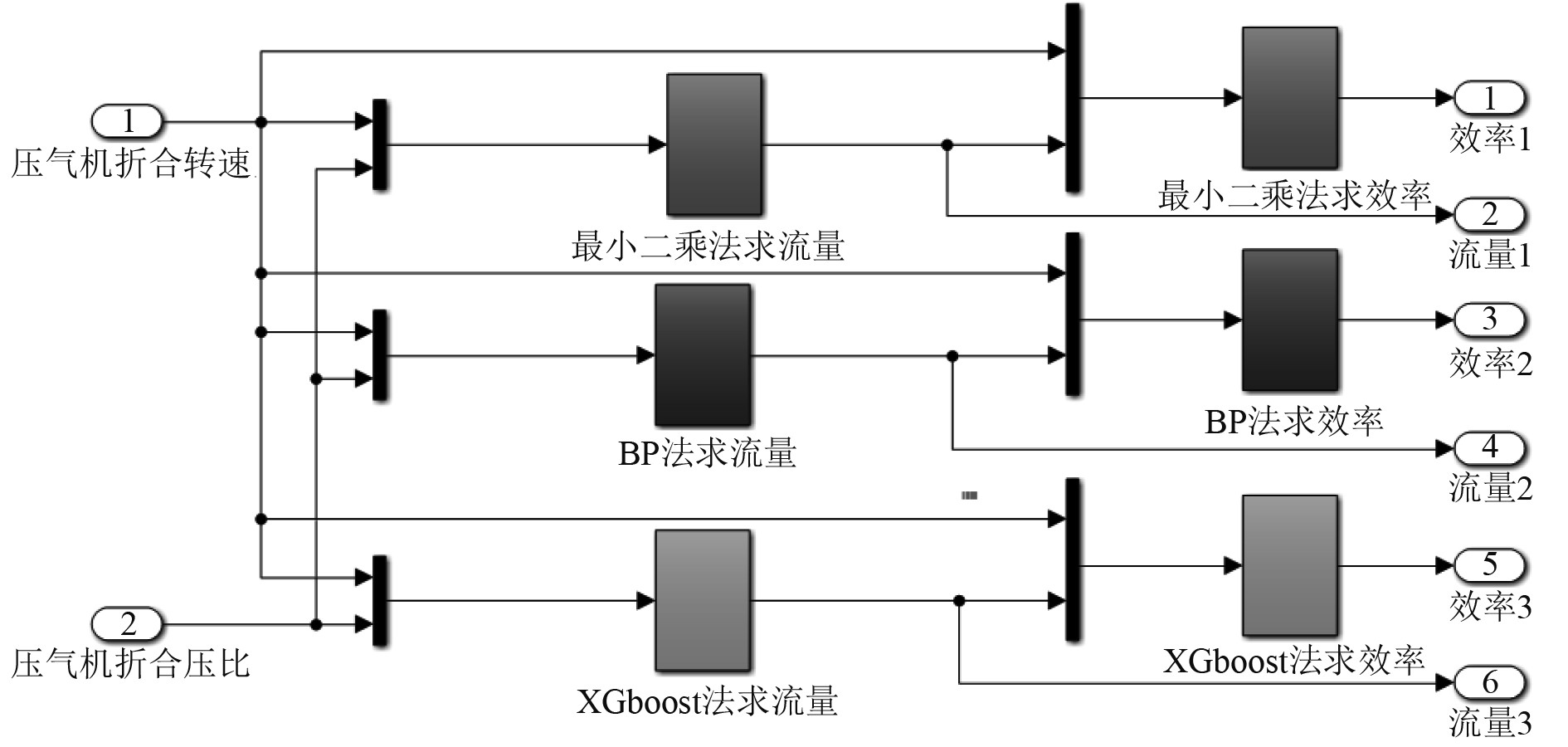图 6 3种预测方法的仿真图 Fig. 6 Simulation diagram of three prediction methods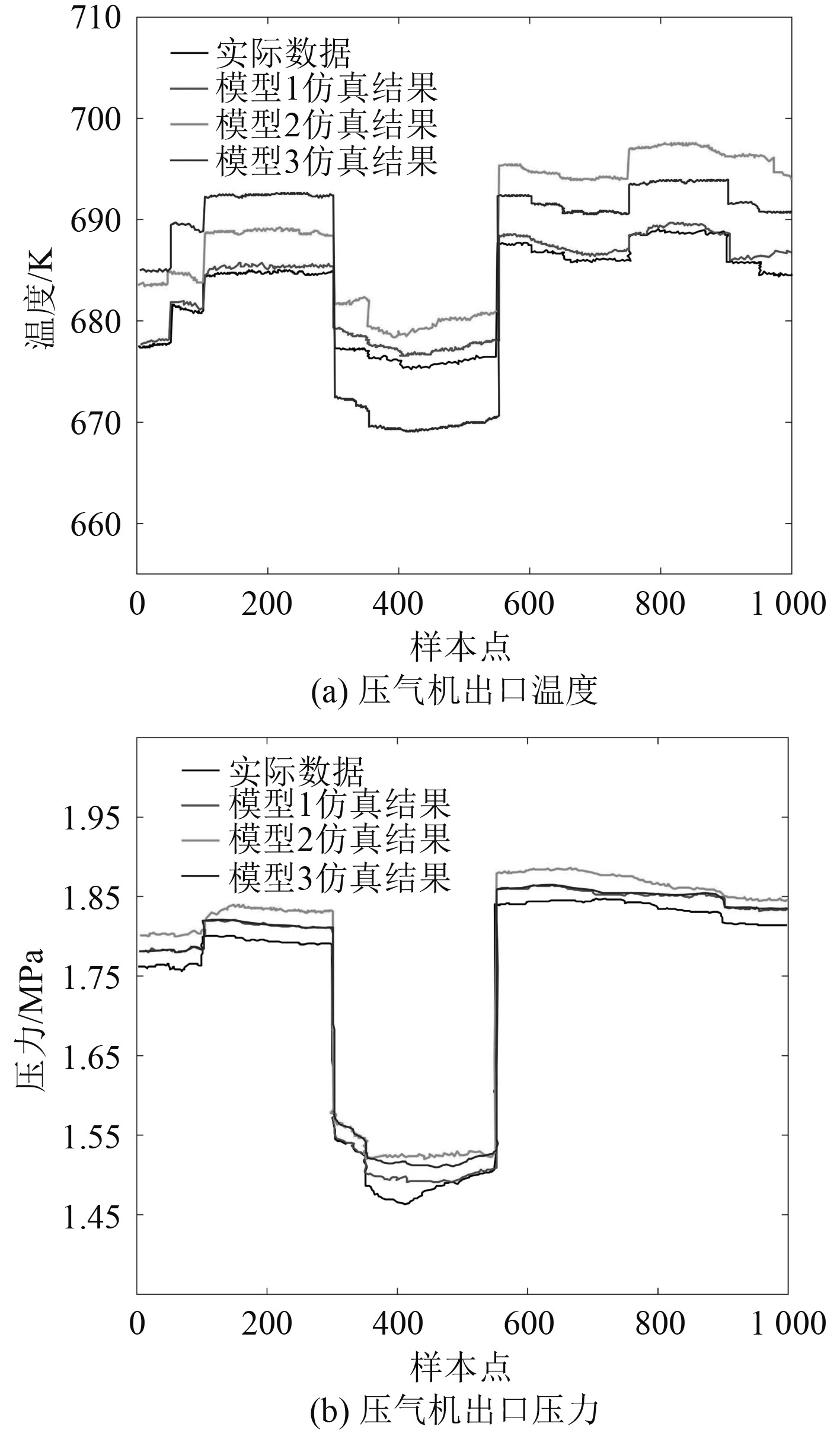图 7 压气机模块仿真 Fig. 7 Compressor module simulation图 8 透平模块仿真 Fig. 8 Turbine module simulation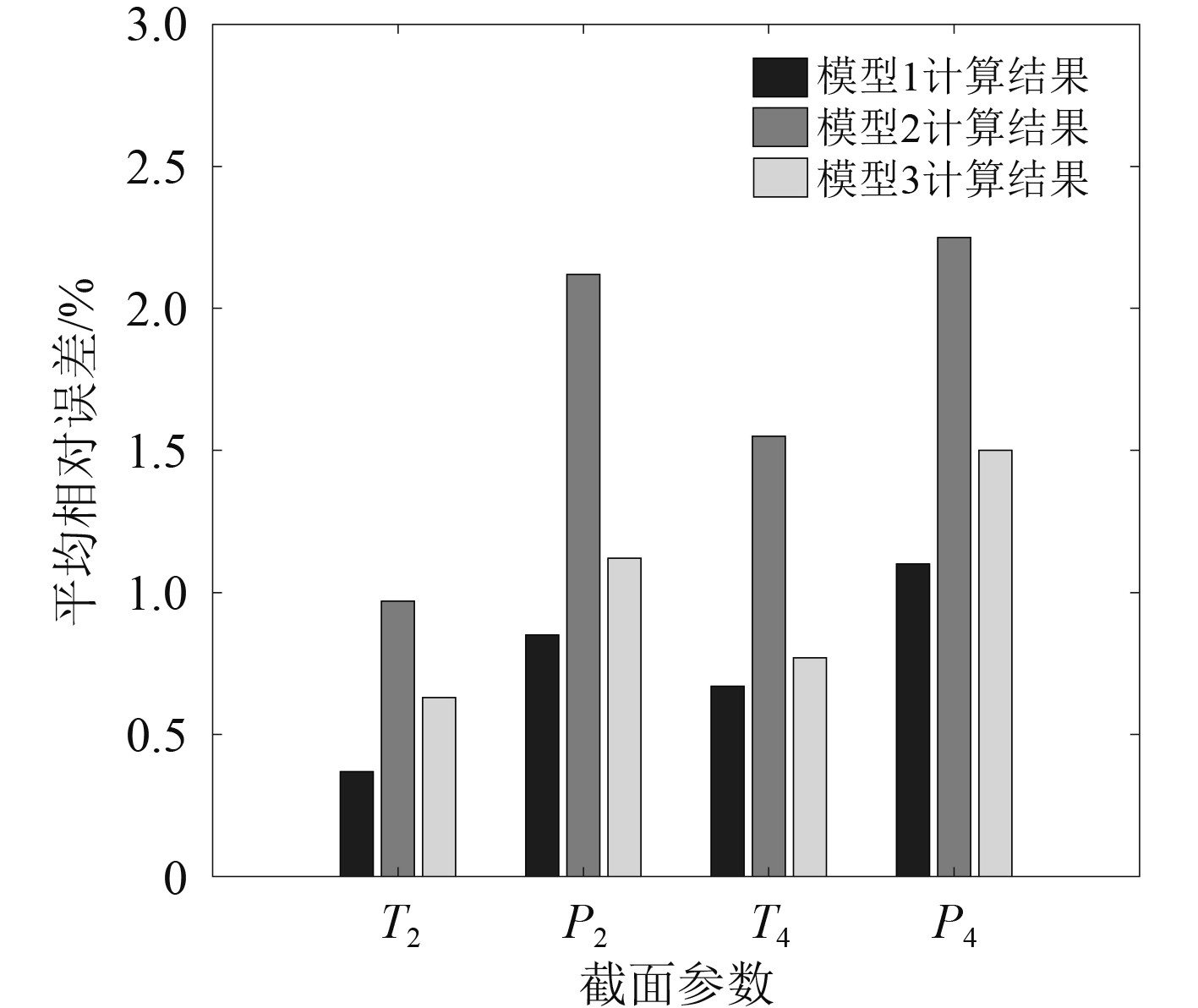图 9 三种模型的平均相对误差 Fig. 9 Average relative error of three models

T2P2T4P4分别是压气机出口温度、压气机出口压力、动力透平出口温度、高压透平出口压力。从图9可以看出，模型1的平均相对误差只有1项是超过1%的，其余平均相对误差都在1%以下。仿真的总体效果表明，模型1的计算结果更加接近实际运行数据。不管是在不同工况下，还是针对不同截面参数的计算，模型1都能够精确地跟随实际运行数据变化趋势。

4 结　语

1）分别利用最小二乘法、BP神经网络法、XGboost算法对燃气轮机压气机特性曲线进行拟合，并比较3种方法拟合数据的相对误差。结果表明3种方法对压气机特性曲线的拟合都能够达到足够的精度，但是BP神经网络法和XGboost算法的计算精度明显要高于最小二乘法。